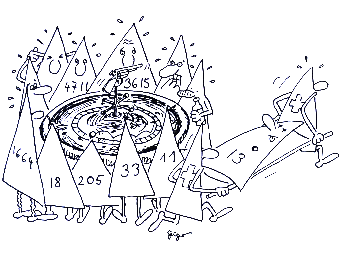# Algorithmic techniques

## Randomized Algorithms

Randomized algorithms are algorithms making random choices.

As opposed to probabilistic algorithms (so-called Monte Carlo algorithms) randomized algorithms (also known as Las Vegas algorithms) give the exact solution of a deterministic problem and do not make any statistic assumption on the input data. Only their complexity depends on the random choices, and is evaluated as a probablistic expectation. Since the undesirable configurations are given a negligible statistic weight, randomization leads to simple and efficient algorithms, namely in geometry.

Randomization also became a method for proving combinatorial results. Finally, as a paradox, derandomizing randomized algorithms yields, in some cases, optimal deterministic algorithms.

 The Prisme group particularly studied incremental randomized algorithms, and developed the Influence Graph. The Influence Graph is a data structure keeping the history of the construction of a given object. Using such a structure leads to semi-dynamic algorithms, able to insert on-line data that are not known in advance. For most fundamental problems, even a fully dynamic algorithm can be obtained, dealing with insertions as well as deletions of data.Another use of randomized techniques was recently done for searching neighbors in very high dimensional spaces in molecular algorithmic.

## Sensitive Algorithms

Sensitive algorithms are algorithms whose complexity depends on the real value of some significative parameters (for instance the size of the computed result) and not on the worst case value of these parameters.

For most geometric problems, the size of the result varies a lot, not only as a function of the number of input data, but also as a function of the configuration of the input data. For instance, the convex hull of a set of n points in dimension d is a polytope, whose numebr of faces can range from (d+1) ! (for a (d+1)-simplex) to $\Omega (n^ { \lfloor {\frac{d+1}{2}} \rfloor })$ (for a maximal polytope with n vertices); the number of intersections of a set of n segments in the plane can range from 0 to n (n-1) / 2. So, for these exemples, it is particularly interesting to have sensitive algorithms whose complexity depends on the size of the result. In other problems, other parameters can play a crucial role. The contribution of the Prisme group in this field essentially relates to convex hull and piercing problems (or of coverage, by duality).

 Last modified: Mon Nov 29 11:09:39 MET 1999 Monique Teillaud TopicsFrançais